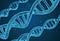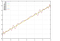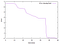# Evolutionary algorithms for optimization (including Java code and CI)The descriptive representation of an object as done by the DNA is the key concept behind evolution.

# Introduction

In this post, I want to introduce you to a kind of algorithm with whom many people have no experience. The pattern of evolutionary algorithms uses the elementary building blocks of natural evolution to find a solution to an optimization problem similar to the way nature optimizes creatures to be able to survive.

An evolutionary algorithm always begins with a set of candidate solutions. In nature, this would be a group of animals. Then, we start a loop that we repeat until we find a candidate that fulfills our requirements. In nature, this loop is still running.

The loop consists of the steps:

• Recombination: The next generation of candidates consists of copies and some candidates, who share properties of multiple original candidates.
• Diversification: To make sure we remain open to new ideas, we regularly add completely new, randomly generated candidates.
• Mutation: We make random changes to the candidates.
• Evaluation /Selection: We judge all the candidates on how well they solve the given problem. In nature, this would be survival. If you live long enough to have offspring, you were successful.
• Restart the loop.

One key difference between EA for mathematical optimization and evolution in nature is that in the optimization case, we make sure to protect the best candidate from mutation or make copies and mutate those. That way, we can ensure with each step, our solution either gets better or stays the same but never deteriorates.

# Genome

One building block is the concept of the genome, the blueprint of an organism. In humans, the DNA stores the genome. The DNA knows two different letters spelling an extensive blueprint of how the human looks. A certain part of the DNA, for example, is used to describe the color of hair. While the DNA stores a blueprint or genotype, the outcome of the blueprint is called the phenotype.

The example problem we will tackle in this article is finding a function that contains certain, given points. For example, the graph of the function f(x) = x² contains the points (2,4) (i.e. f(2) = 4), (0,0) and (1,1). While it is easy to compute the points, a function graph contains, it is tough to determine the function from a given set of points. For simple functions, we might be able to identify a function from the shape of the plot. Still, as soon as the function contains non-integer factors, this can be hard, and if there is some disturbance to the signals (like for inaccuracies in measurement), it is impossible.

# Application of the EA-pattern to the example problem

First, we assume that we have a set of points or measurements, for whom we know the value of the function exactly. Then we assume we have a language to describe all possible building blocks of mathematical functions. We can use this language to come up with some random mathematical expressions. These could be

f(x) = x ⋅ x+ 0.5,

f(x) = (x + 2)⋅ x, and

f(x) = x².

That concludes step 1. The points as mentioned above are (2,4), (0,0) and (1,1). Evaluation can be done by simply evaluating the functions for the given values of x. We can then compute how far off the functions are. We add all these distances up for every individual function and have a fitness value for each function.

The sums of differences for each function describe the fitness of the functions — or, to be more precise, the “unfitness” since 0 here is the optimal value. So, in this case, we would already be done since we have found the perfect candidate.

If we hadn’t found that perfect solution right away, we would now have to recombine the functions, mutate them, and then reevaluate them. Selection is only required to limit the number of candidates — an increasing number of candidates would slow down the other steps over time.

Mutation can be done by simply altering some of the parts. We can change +0.5 in f₁ to +0.6. Recombination can be done by exchanging parts between functions. As an example, we could replace x ⋅ x in f₁ by f₂, which would result in f₁ ₂(x) = (x + 2) ⋅ x +0.5.

So, in this case, we have found the worst function so far. However, this example describes how an algorithm for this problem would work so we can move on to details about the implementation. For this example, I have decided to go with Java as a programming language. Object orientated programming and good IDEs were the reason to go with Java (and Eclipse) for a fast prototype.

# Implementation

First, we implement a basic data type called Expression that describes the properties of a mathematical term and the primary mechanisms for mutation. For this example, I have decided to only go with mutation between generations instead of also implementing recombination, which would likely speed up the convergence towards a solution, but that is not our primary concern now. An Expression is supposed to have the following properties:

• Evaluate for a given value of x.
• Be cloneable, which enables us to create copies for later generations.
• Be able to mutate so that we can perform a small change on the object.
• It should have a list of child expressions. The expression could, for example, be a sum. In that case, it would have two child expressions. One left of the + and one to the right. A sinus function would only have one child expression — the term inside the brackets.
• A depth. If an expression can have multiple children, this could lead to an infinite number of expressions unless the depth was limited somehow. The final building-blocks would be the two kinds of expressions without any children: Either a constant number or a variable.

These properties we put into an abstract base class called Expression, from which we then derive the classes Constant, Multiplication, SpecialFunction, Sum, and VariableValue. The SpecialFunction type contains logic to turn into sin(x), cos(x), exp(x), and the square root of x.

Additionally, we require an object that will run the parts of the evolutionary process, i.e., create expressions, evaluate them, mutate and recombine them as well as select the ones that go to the next generation. It contains a loop that performs these steps and, in the end, checks if one of the candidates is close enough to the expected solution.

The runner should also write output to help supervise the optimization process and to check for mistakes in the code. We can also use it to write detailed output like function plots or graphics to visualize the candidates. A mathematical expression, for example, can be visualized as a tree of expressions.

These kinds of programs always depend strongly on a lot of parameters.

• What is a good starting set?
• How many candidates are there at any given time?
• How many survive a generation?
• How strongly do they mutate?
• Where do they mutate?
• How deep can the expressions be packed?

For evolutionary algorithms, a perfect implementation is difficult since it depends strongly on random (for example, the random candidates to start with). Additionally, there are no exact solutions. A version with recombination, for example, is better than one without. However, one without recombination can also yield good results. So even if we implement all necessary parts, there can still be good or bad implementations, independent of the quality of the code. Evolutionary Algorithms require finetuning and have to be tailored to the problem.

One more point to consider is the following: In some sense, the mathematical terms created in this example are only as good as the sum of their parts. So if, for example, only expressions for sum, product, and constants are implemented (and no dependency on a variable — i.e., the term x), then all possible functions are constant functions. Or if there are only terms x, constant numbers, and sums, then all possible functions are of the form f(x) = ax + b. It is, therefore, essential to have enough complexity in the building blocks to approximate complex functions.

Also, a mechanism to simplify functions is useful in this example. The term 3 + 7, for example, can be reduced to the constant expression of 10. Simplification reduces the artificial depth of expressions and frees up space for actual expressions. To implement this, you can implement a function isConstant() that returns true if all parts of this expression are constant — if necessary, checking if the expressions it contains are constant themselves. So ((2 + 5) + x) can be reduced to (7 + x) but not any further because x is not constant and therefore 7+x isn’t either.

# Example output

You can find a complete Java implementation in my Github account. As an example, we consider f(x) = sin(x²) ⋅ cos(x) + x, which we try to approximate with our scheme. We pass the values of the exact function for the x-values -5, -4, -3 up to 5. In the following graph, the numbers in the function name give the generation that function is from, and the function is always the best approximation of its generation. So f(x) is the best approximation in generation 1, f₃₀(x) in generation 30, and so on. f(x) is the plot of the exact function.This plot shows the best members of the generations 1,10,15,30,50 and 60.

We see that the early attempts (f(x) and f₁₀(x)) are straight lines somewhere near the exact solution. In generations 15 and 30, we begin to see oscillation that tries to approximate the correct behavior, and 50 and 60 are so precise they cover the exact graph completely. The next plot shows how the error has evolved across generations:Development of the error of the best approximation in each generation.

# Problems of this method

The error plot shows that we can find a solution to our problem that is very close to the actual law behind the points we used. However, unless we make an effort to simplify the expressions we find, they can become very complex. For example, the function f₆₀(x) looks like this:

f₆₀(x) = (((-0.3096 ⋅ -7.496)⋅ -1.009 ⋅ 0.000001) + x) + (sin(x ⋅ x)) ⋅ cos(x)

If you look at the expression, you can see that the first part is almost exactly x (because the rest gets multiplied by 0.000001), and the part at the end is sin(x²) ⋅ cos(x). The version currently available in my GitHub repository contains logic to remove products and sums of constants to simplify the equation, however, so the result it would find would look nicer.

Generation of the original candidates, mutation, and recombination heavily depend on randomness. To this end, we make extensive use of a random number generator. For that reason, unless you store the seed of the random number generator, multiple runs of the same code will yield different results, which makes statements about efficiency difficult. If we were extremely unlucky, we would never make any progress with our scheme. This, however, is unlikely to happen, and we can make statements about expected outcomes after a certain number of attempts and runtime.

As you can see in the repository at GitHub, the repository does not only contain the code. There are also automated tests that check if the implementation is correct. These tests get executed every time a change is made and the results are shown as a badge. This is a common way of protecting code against regression, i.e. the introduction of errors into code which leads to a loss of functionality. If you click the CircleCI badge, you can see how the tests have developed over the past with every change that has been made to the code. This link will take you there directly. This is a good approach to protect your code and to make sure that small building blocks do exactly what they should.

To determine how well your code is protected by tests, you can compute coverage. Coverage describes if a line of code gets executed in a test or not. Ideally, all your code should be covered and there are tools that show you if it is. The one I have used in this project is CodeCov. The important thing about CircleCI and CodeCov is, that they are free to use with Github repositories, as long as the repository is public. This is a great option for anyone working open source.

This work was also published on my website at light-and-code.com and the code is openly available at GitHub.

## More From Medium

#### More from The Startup

Mar 24 · 5 min read

#### More from The Startup

Mar 29 · 9 min read

#### More from The Startup

Mar 25 · 8 min read

#### 1.7K

Welcome to a place where words matter. On Medium, smart voices and original ideas take center stage - with no ads in sight. Watch
Follow all the topics you care about, and we’ll deliver the best stories for you to your homepage and inbox. Explore
Get unlimited access to the best stories on Medium — and support writers while you’re at it. Just \$5/month. Upgrade